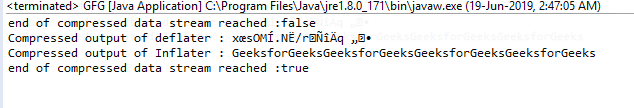# Inflater finished() function in Java with examples

The finished() function of the Inflater class returns true if the end of compression data stream has been reached.

Function Signature:

```public boolean finished()
```

Syntax:

```i.finished();
```

Parameter: The function requires no parameter

Return Type: The function returns boolean value, returns true if all the input is uncompressed and stored in the given buffer, else return false.

Exception: The function does not throw any exception

Example 1: use of finished function

 `// Java program to describe the use ` `// of finished() function ` ` `  `import` `java.util.zip.*; ` `import` `java.io.UnsupportedEncodingException; ` ` `  `class` `GFG { ` `    ``public` `static` `void` `main(String args[]) ` `        ``throws` `UnsupportedEncodingException, ` `               ``DataFormatException ` `    ``{ ` ` `  `        ``// compress the data ` ` `  `        ``// deflater ` `        ``Deflater d = ``new` `Deflater(); ` ` `  `        ``// get the text ` `        ``String pattern = ``"GeeksforGeeks"``, text = ``""``; ` ` `  `        ``// generate the text ` `        ``for` `(``int` `i = ``0``; i < ``4``; i++) ` `            ``text += pattern; ` ` `  `        ``// set the input for deflator ` `        ``d.setInput(text.getBytes(``"UTF-8"``)); ` ` `  `        ``// finish ` `        ``d.finish(); ` ` `  `        ``// output bytes ` `        ``byte` `output[] = ``new` `byte``[``1024``]; ` ` `  `        ``// compress the data ` `        ``int` `size = d.deflate(output); ` ` `  `        ``// end ` `        ``d.end(); ` ` `  `        ``// end of compression ` ` `  `        ``// use Inflater to get back the original data ` ` `  `        ``// Inflater ` `        ``Inflater i = ``new` `Inflater(); ` ` `  `        ``// value returned by finished function ` `        ``System.out.println(``"end of compressed data stream reached :"` `                           ``+ i.finished()); ` ` `  `        ``// set the input for inflator ` `        ``i.setInput(output); ` ` `  `        ``// output bytes ` `        ``byte` `inflater_output[] = ``new` `byte``[``1024``]; ` ` `  `        ``// uncompress the data ` `        ``int` `org_size = i.inflate(inflater_output); ` ` `  `        ``// output of inflater and deflater ` `        ``System.out.println(``"Compressed output of deflater : "` `                           ``+ ``new` `String(output)); ` `        ``System.out.println(``"Compressed output of Inflater : "` `                           ``+ ``new` `String(inflater_output, ``"UTF-8"``)); ` ` `  `        ``// value returned by finished function ` `        ``System.out.println(``"end of compressed data stream reached :"` `                           ``+ i.finished()); ` ` `  `        ``// end ` `        ``i.end(); ` `    ``} ` `} `

Output:My Personal Notes arrow_drop_upCheck out this Author's contributed articles.

If you like GeeksforGeeks and would like to contribute, you can also write an article using contribute.geeksforgeeks.org or mail your article to contribute@geeksforgeeks.org. See your article appearing on the GeeksforGeeks main page and help other Geeks.

Please Improve this article if you find anything incorrect by clicking on the "Improve Article" button below.

Article Tags :
Practice Tags :

Be the First to upvote.

Please write to us at contribute@geeksforgeeks.org to report any issue with the above content.